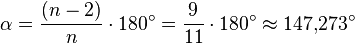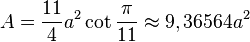# Hendecagon facts for kids

Kids Encyclopedia Facts
(Redirected from Hendecagram)

A hendecagon or 11-gon is a shape with 11 sides and 11 corners.

## Regular hendecagon

All sides of a regular hendecagon are the same length. Each corner is 147.27°. All corners added together equal 6840°.

### Area

The amount of space a regular hendecagon takes up is$\alpha = \frac{(n - 2)}{n} \cdot 180^\circ = \frac{9}{11} \cdot 180^\circ \approx 147{,}273^\circ$$A = \frac{11}{4}a^2 \cot \frac{\pi}{11} \approx 9,36564a^2 \,$

a is the length of one of its sides.

## Images for kidsHendecagon Facts for Kids. Kiddle Encyclopedia.